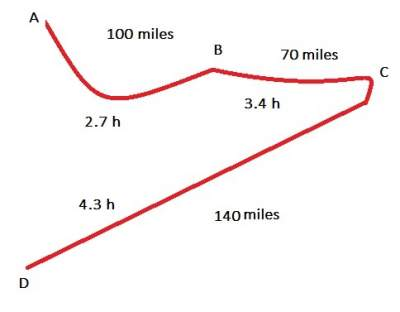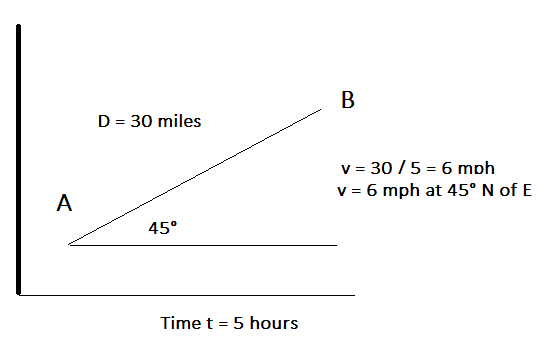# Speed and Velocity

## Introduction

Just as distance and displacement have distinctly different meanings, so do speed and velocity.
Speed is a scalar quantity that refers to "how fast an object is moving."
Speed can be thought of as the rate at which an object covers distance and its formula is: v = d/t where d is the distance and t is time.
Example: Assume that Mike took him 2 hours minutes to walk 6 miles. What is his speed in miles per hour (mph) during this exercise?
Answer: v = 6 / 2 = 3 mph.

## Exercise: Unit Conversion

### Exercise 1:

Question: Convert 80 kilometers per hour to miles per hour. Solution:
1 km = 0.6214 miles; 1 mile = 1.609 km
80 km/h × 0.6214 = 49.71 mph

### Average Speed

Average Speed = Distance Traveled / Time of Travel

### Exercise 2:

Question: Calculate the average speed of a Taxi driver who started a trip from point A to D passing by B and C with speed mentioned in the figure below:#### Exercise

Adam drove for 4 hours at a rate of 70 miles per hour and for 3 hours at 55 miles per hour.
Question: What was his average speed for the entire trip?

### Velocity

Velocity is a vector quantity that refers to "the rate at which an object changes its position."
As depicted below the velocity requires direction:### Average Velocity

Average Velocity = Displacement / Time = ΔPosition / Δt = Δd / Δt
Note the difference between distance and displacement.
• Displacement is "how far out of place an object is" it is the object's overall change in position.
• The speed at any instant is called instantaneous speed.
• The speedometer in the car gives instantaneous speed.

#### Exercise

Let's assume that Joe while jogging, during the first two hours of his exercise,
he ran a distance from work started from 0 to 8 miles, while the time in hours went from 0 to 2.
Question 1: Calculate his average velocity.

Question 2:
During the next three hours, the distance from his work went from 8 to 23 while the time went from 1 to 4.
Calculate his average velocity.

Check both answers here: Average Velocity Exercise